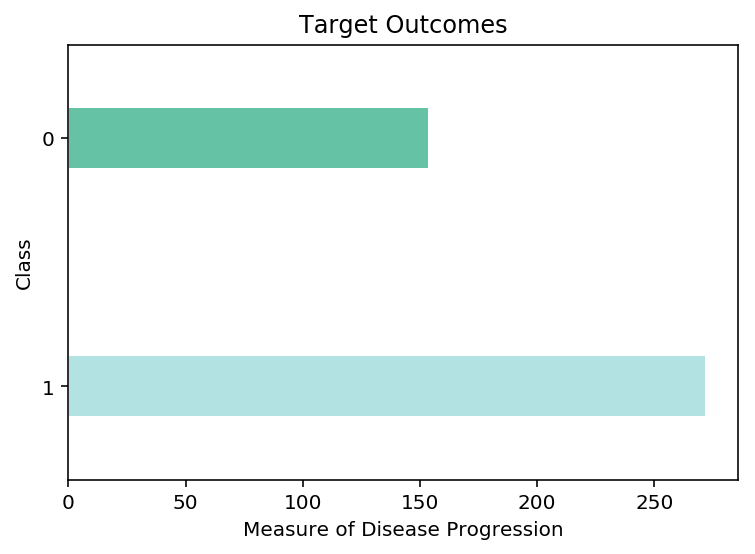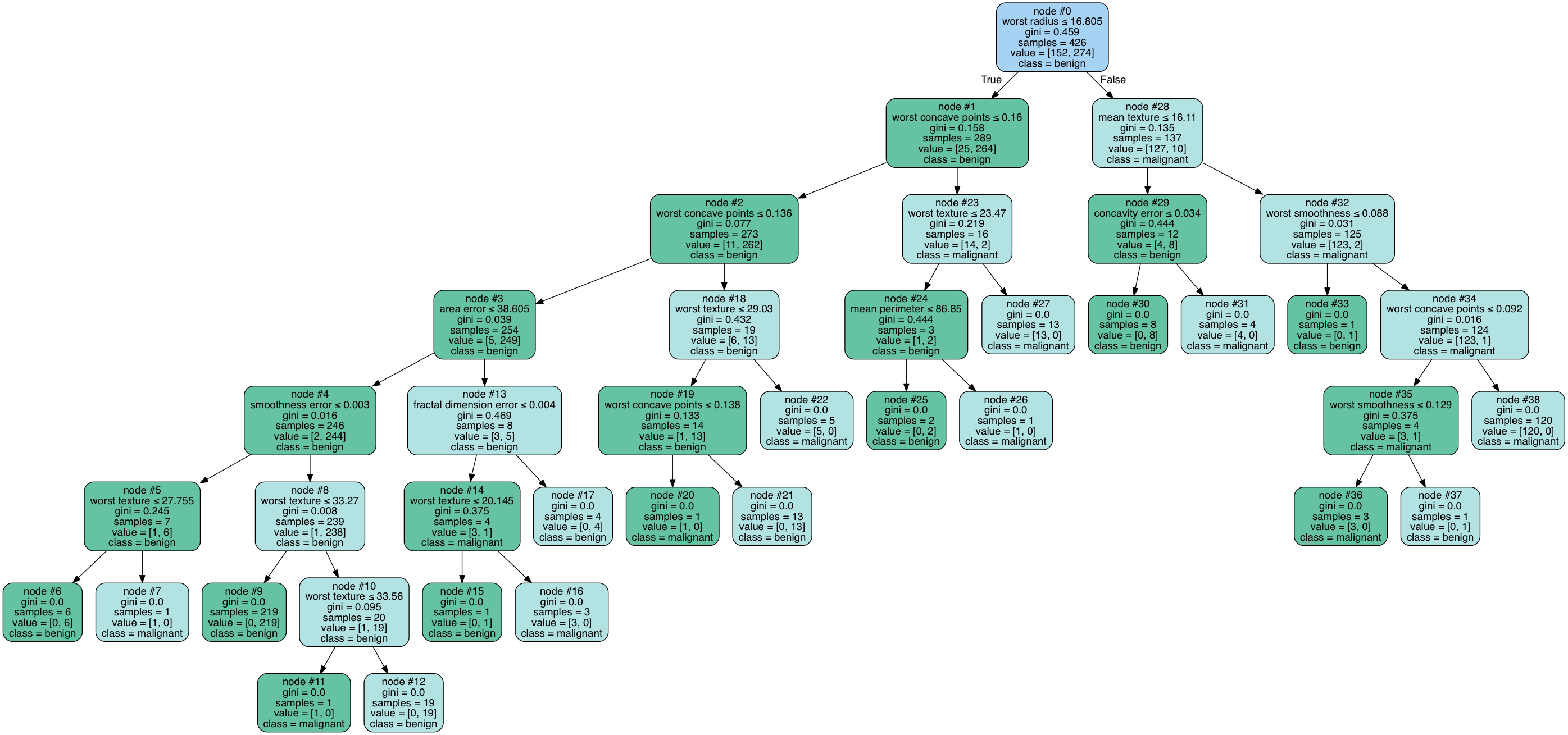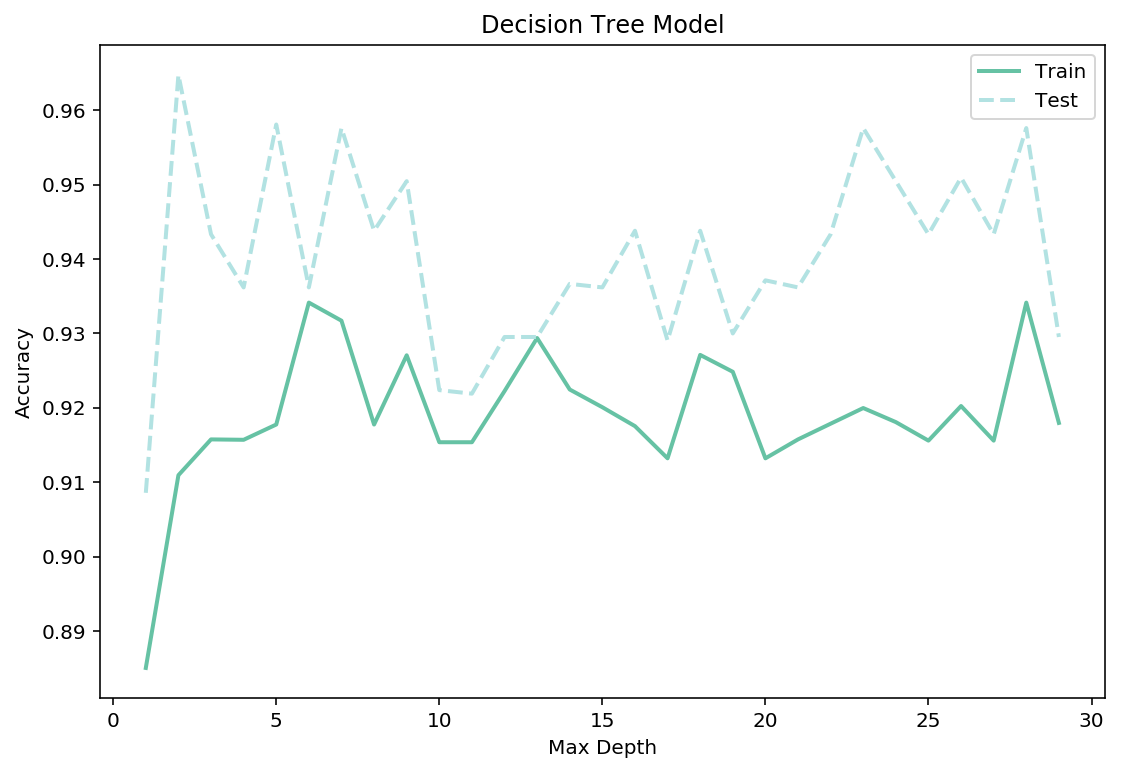01 Sep

## Decision Tree Classifier

A decision tree classier is a straightforward tree-like model. The classifier is just a decision tree and split the classes on each layer via a heuristics. The methodology is both used in machine learning and operation research. Using the Sklearn, the model can tuned with various hyperparameters performance.

In this notebook, I will be looking at the famous breastcancer dataset. This dataset is a multi-class classification problem, where I need to predict the correct target for each observation from a range of possible classes. We will attempt to predict the proper target class using this model, given the feature of each type of class, I often reuse this dataset between my tree-based notebooks. Using the same dataset makes it very easy to compare and contrast the performance of different tree-based models, and keep the trees a reasonable size.

Dataset

Breast Cancer Dataset: https://www.kaggle.com/hdza1991/breast-cancer-wisconsin-data-set

### Import Preliminaries¶

In :
%matplotlib inline
%config InlineBackend.figure_format='retina'

# Import modules
import collections
import graphviz
import matplotlib.pyplot as plt
import matplotlib as mpl
import numpy as np
import pandas as pd
import pydotplus
import warnings

from IPython.display import Image
from sklearn.externals.six import StringIO
from sklearn.model_selection import train_test_split, cross_val_score, GridSearchCV
from sklearn.tree import DecisionTreeClassifier, export_graphviz
from sklearn import tree

# Set pandas options
pd.set_option('max_columns',1000)
pd.set_option('max_rows',30)
pd.set_option('display.float_format', lambda x: '%.3f' % x)

# Set plotting options
mpl.rcParams['figure.figsize'] = (9.0, 3.0)

# Set warning options
warnings.filterwarnings('ignore');


### Import Data¶

In :
# Import Breast Cancer data
X, y = breast_cancer.data, breast_cancer.target

# Conduct a train-test split on the data
train_x, test_x, train_y, test_y = train_test_split(X,y)

# View the training dataframe

Out:
mean radius mean texture mean perimeter mean area mean smoothness mean compactness mean concavity mean concave points mean symmetry mean fractal dimension radius error texture error perimeter error area error smoothness error compactness error concavity error concave points error symmetry error fractal dimension error worst radius worst texture worst perimeter worst area worst smoothness worst compactness worst concavity worst concave points worst symmetry worst fractal dimension
0 17.540 19.320 115.100 951.600 0.090 0.120 0.104 0.075 0.151 0.055 0.397 0.828 3.088 40.730 0.006 0.026 0.027 0.013 0.016 0.003 20.420 25.840 139.500 1239.000 0.138 0.342 0.351 0.194 0.293 0.079
1 13.300 21.570 85.240 546.100 0.086 0.064 0.033 0.024 0.181 0.057 0.262 1.539 2.028 20.980 0.005 0.020 0.018 0.006 0.018 0.002 14.200 29.200 92.940 621.200 0.114 0.167 0.121 0.056 0.264 0.067
2 13.590 21.840 87.160 561.000 0.080 0.083 0.041 0.021 0.164 0.059 0.338 1.916 2.591 26.760 0.005 0.024 0.031 0.010 0.020 0.003 14.800 30.040 97.660 661.500 0.101 0.173 0.145 0.062 0.245 0.070
3 10.490 19.290 67.410 336.100 0.100 0.086 0.030 0.012 0.222 0.065 0.355 1.534 2.302 23.130 0.008 0.022 0.029 0.009 0.027 0.003 11.540 23.310 74.220 402.800 0.122 0.149 0.080 0.032 0.283 0.076
4 15.780 17.890 103.600 781.000 0.097 0.129 0.100 0.066 0.184 0.061 0.506 0.985 3.564 54.160 0.006 0.041 0.028 0.013 0.020 0.004 20.420 27.280 136.500 1299.000 0.140 0.561 0.397 0.181 0.379 0.105
In :
# Plot a barplot of the target clasees
pd.Series(train_y).value_counts().plot.barh(grid=False, color=['#B2E2E2','#66C2A4'], width=0.25,edgecolor='w')
plt.title('Target Outcomes')
plt.ylabel('Class')
plt.xlabel('Measure of Disease Progression');### Fit the Model¶

In :
# Fit the intial model
dt_model = DecisionTreeClassifier()
dt_model.fit(train_x, train_y);


### Model Evaluation¶

##### Cross Validation Score¶
In :
# View the cross validation score of the intial model
scores = cross_val_score(dt_model, train_x, train_y, cv=10,
scoring='accuracy')
print(f'Cross Validation Score: {scores.mean():.5f}')

Cross Validation Score: 0.95325


#### Confustion Matrix¶

In :
# Training Confusion Matrix
from sklearn.metrics import confusion_matrix
cmatrix = pd.DataFrame(confusion_matrix(train_y, dt_model.predict(train_x)))
cmatrix.index.name = 'class'

cmatrix['result'] = 'actual'
cmatrix.set_index('result', append=True, inplace=True)
cmatrix = cmatrix.reorder_levels(['result', 'class'])
cmatrix = cmatrix.stack()
cmatrix = pd.DataFrame(cmatrix)
cmatrix.columns = ['prediction']
cmatrix.unstack()

Out:
prediction
0 1
result class
actual 0 152 0
1 0 274
##### Tree Diagram¶
In :
dot_data = StringIO()

# Export graph from sklearn
export_graphviz(dt_model, out_file=dot_data,
filled=True, rounded=True,
special_characters=True,
feature_names = breast_cancer['feature_names'],
class_names = breast_cancer['target_names'],
node_ids = True, proportion= False)

# Generate graphusing pydotplus
graph = pydotplus.graph_from_dot_data(dot_data.getvalue())

# Color Decision Tree
colors = ('#66C2A4', '#B2E2E2')
edges = collections.defaultdict(list)
for edge in graph.get_edge_list():
edges[edge.get_source()].append(int(edge.get_destination()))

for edge in edges:
edges[edge].sort()
for i in range(2):
dest = graph.get_node(str(edges[edge][i]))
dest.set_fillcolor(colors[i])

# Save Image
graph.write_png('Images/dt_model.png')

# View Decision Tree Plot
Image(graph.create_png())

Out:##### Parameter Tuning¶
In :
# Define paraameter range and score lists
max_depth_range = np.arange(1,30)
train_score = []
test_score = []

# Train a knn_model for every neighbour value in our list
for i in max_depth_range:
dt_model=DecisionTreeClassifier(max_depth = i).fit(train_x,train_y)
train_score.append(cross_val_score(dt_model, train_x, train_y, cv=10, scoring='accuracy').mean())
test_score.append(cross_val_score(dt_model, test_x, test_y, cv=10, scoring='accuracy').mean())

# Plot our results
mpl.rcParams['figure.figsize'] = (9.0, 6.0)
plt.plot(max_depth_range,train_score,label="Train",linewidth=2, color='#66C2A4')
plt.plot(max_depth_range,test_score,label="Test", linewidth=2,linestyle='--', color='#B2E2E2')
plt.legend()
plt.title('Decision Tree Model')
plt.xlabel('Max Depth')
plt.ylabel('Accuracy');##### Feature Importance¶
In :
# Plot Tree's Feature Importance
n_features = breast_cancer.data.shape
plt.barh(range(n_features), dt_model.feature_importances_, align='center', color='#4D977E')
plt.yticks(np.arange(n_features), breast_cancer.feature_names)
plt.title('Decision Tree Feature Importance')
plt.xlabel("Feature importance")
plt.ylabel("Features")
plt.ylim(-1, n_features);